### Home > MC1 > Chapter 6 > Lesson 6.2.2 > Problem6-60

6-60.
1. . Find each of the parts of parts described below. For each one, create a diagram that demonstrates your thinking. Homework Help ✎

1.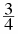of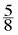2.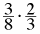3.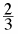of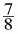4.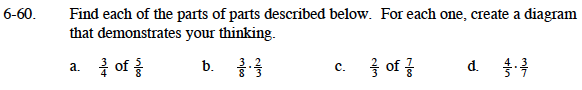Draw a diagram to represent five eighths.

Split five-eighths into fourths.

$\frac{15}{32}$$\frac{6}{24}=\frac{1}{4}$

Draw a diagram like you did for part (a). This will help you visualize the part of a fraction.

Follow the steps in part (a).

Follow the steps in part (a).